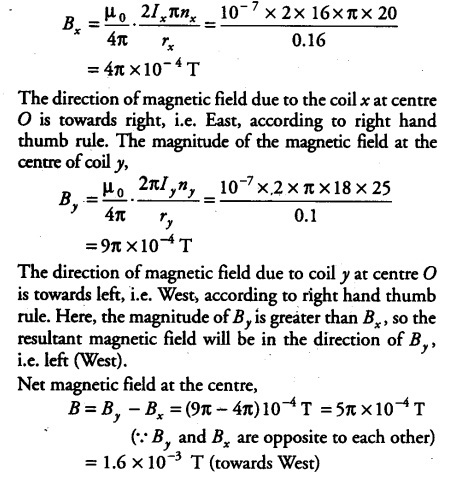# Wo concentric circular coils x and y of radii 16 cm and 10 cm respectively

Two concentric circular coils x and y of radii 16 cm and 10 cm respectively lie in the same vertical plane containing the North to South direction. Coil x has 20 turns and carries a current of 16 A, coil y has 25 turns and carries a current of 18 A . The sense of the current in x is anti-clockwise and clockwise in y, for an observer looking at the coils facing West. Find the magnitude and direction of the net magnetic field due to the coils at their centre.

For coil x
Radius of coil, { r }_{x } = 16 cm = 0.16 m
Number of turns, { n }_{x } = 20
Current in the coil, { I }_{x } = 16 A (anti-clockwise)For coil y
Radius of coil, { r }_{ y }= 10 cm =0.1 m
Number of turns, { n }_{ y } = 25
Current in the coil, I = 18 A (clockwise)
The magnitude of the magneticfield at the centre of coil x